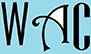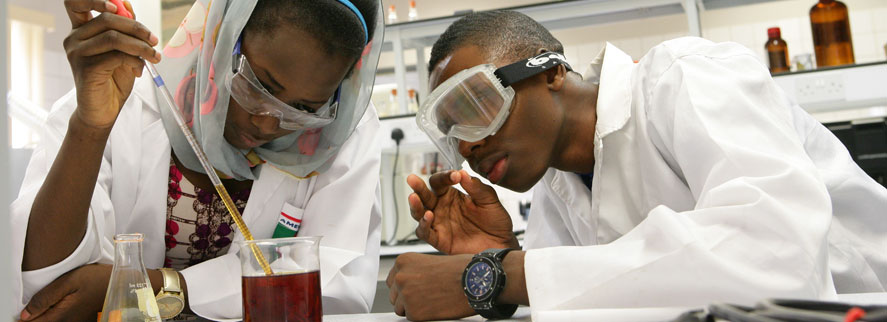## Geometry Question for Title 1 Schools: Circle Area & Diameter Question?

7) What is the diameter and area of a circle with a circumference of 6.28?

Circumference “c” = 2пr, Area of a circle “a” = пr2, where п = 3.14and r = radius (half of a diameter “D”)

c = 2пr, so to find “r”, you have to make “r” subject of formula by moving “2п” to the other side. when you go to the other side, addition becomes subtraction, multiplication becomes division, and vice versa.

Since “2п” is a multiplication to “r”, it will become a division at the other side

c/ 2п = r

6.28/ (2 x 3.14) = r

6.28/6.28 = r

1 = r; so if the radius  “r” = 1, then the diameter “D” = 2 (since radius is half of diameter 1 x 2 = 2)

a = пr2

a = 3.14 x 12

so the area of the circle = 3.14